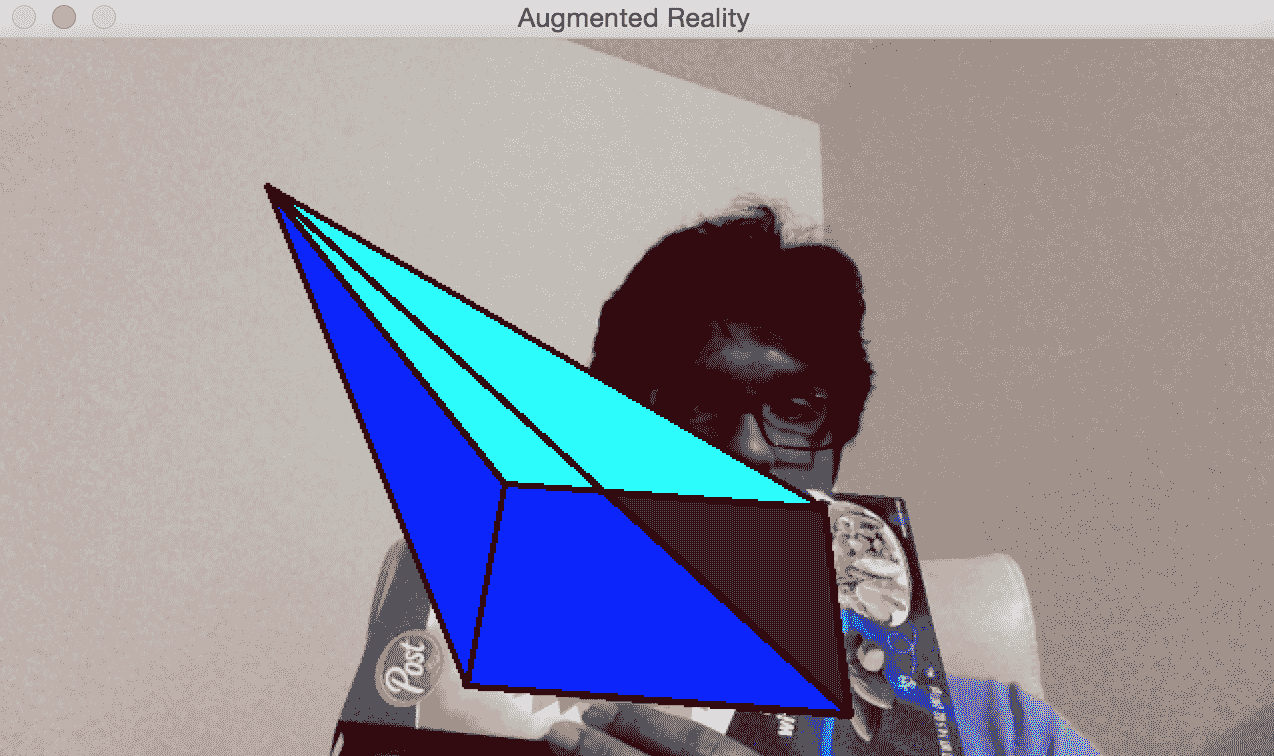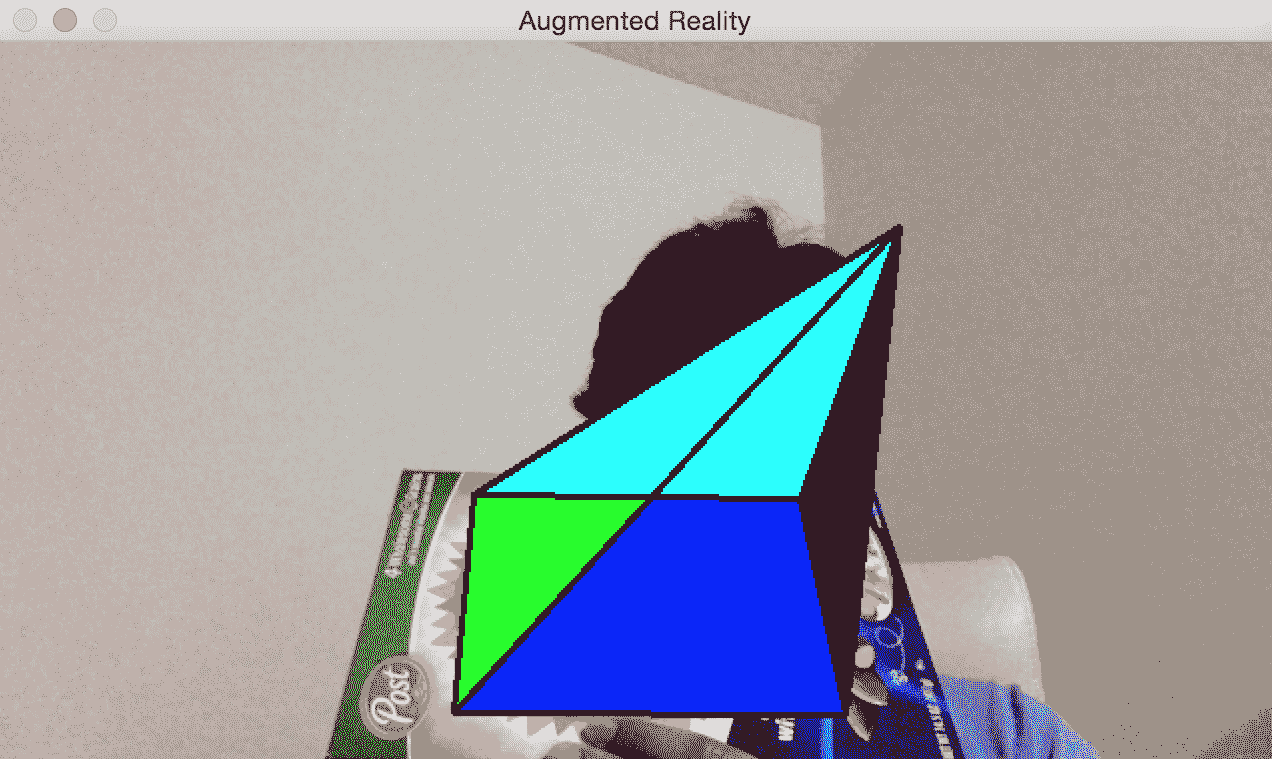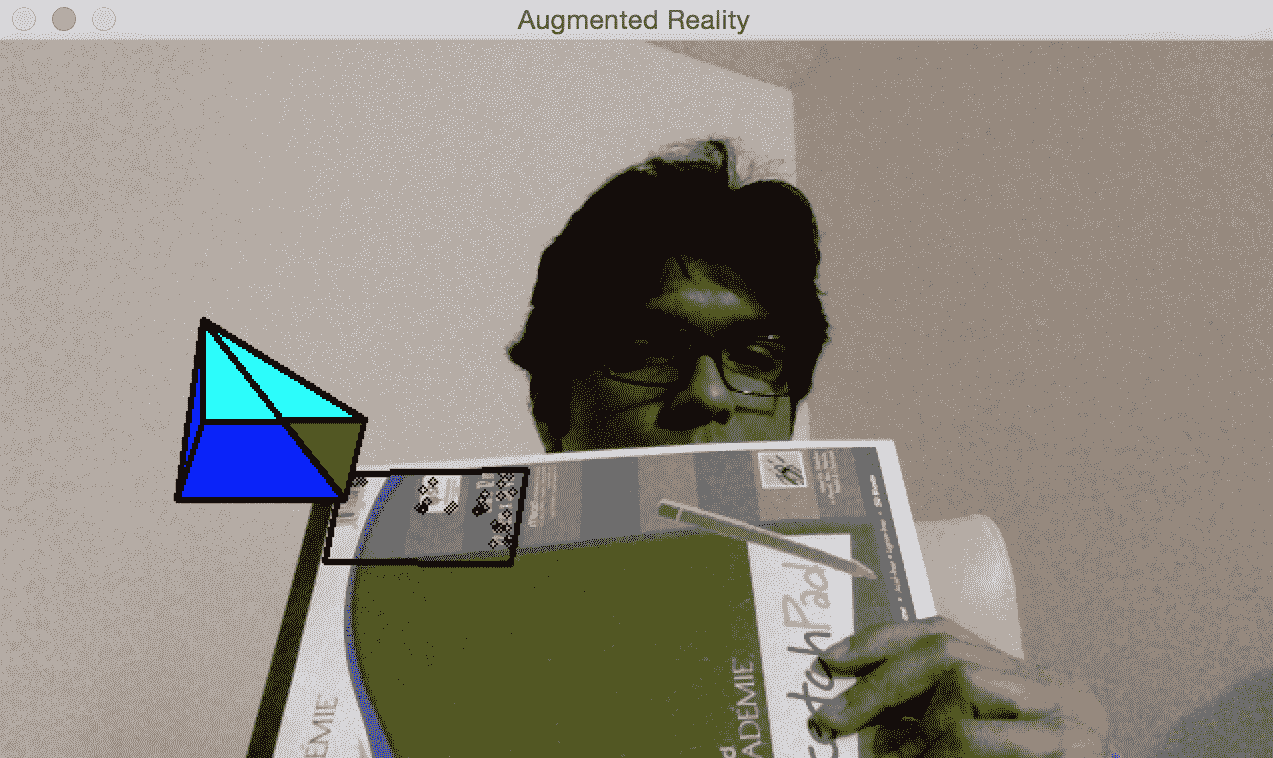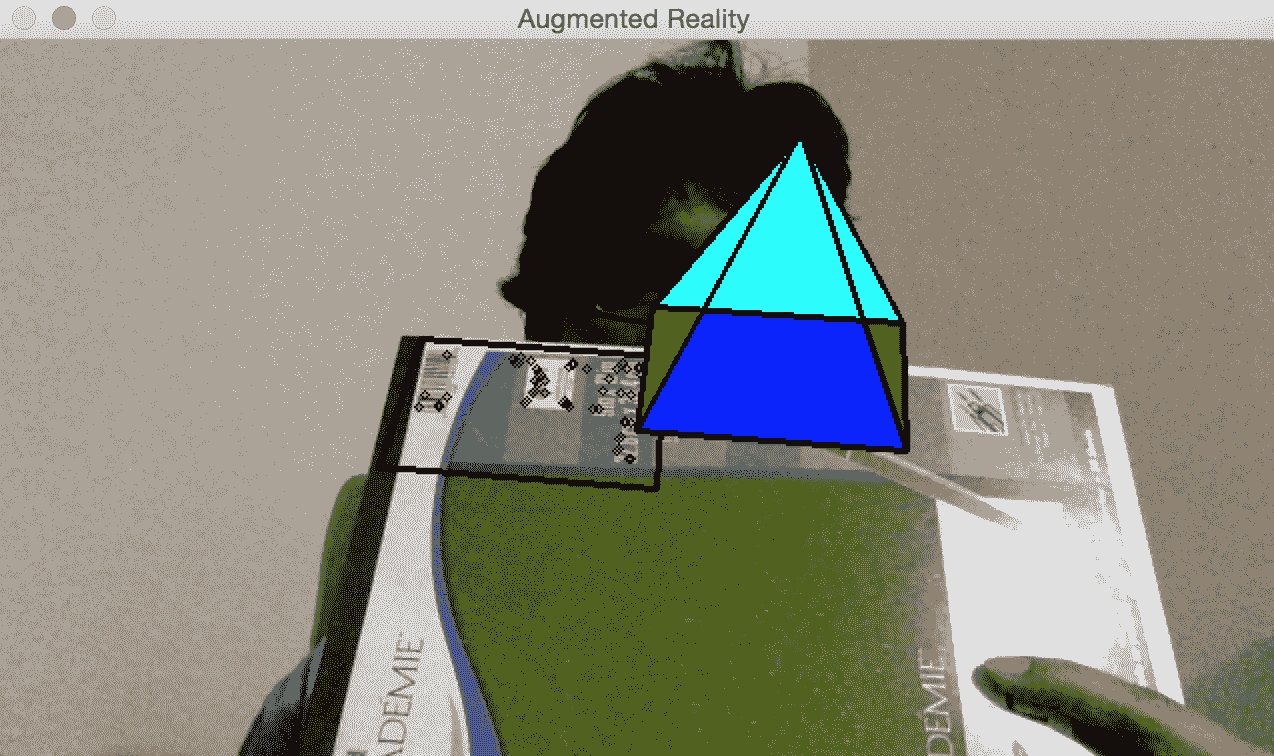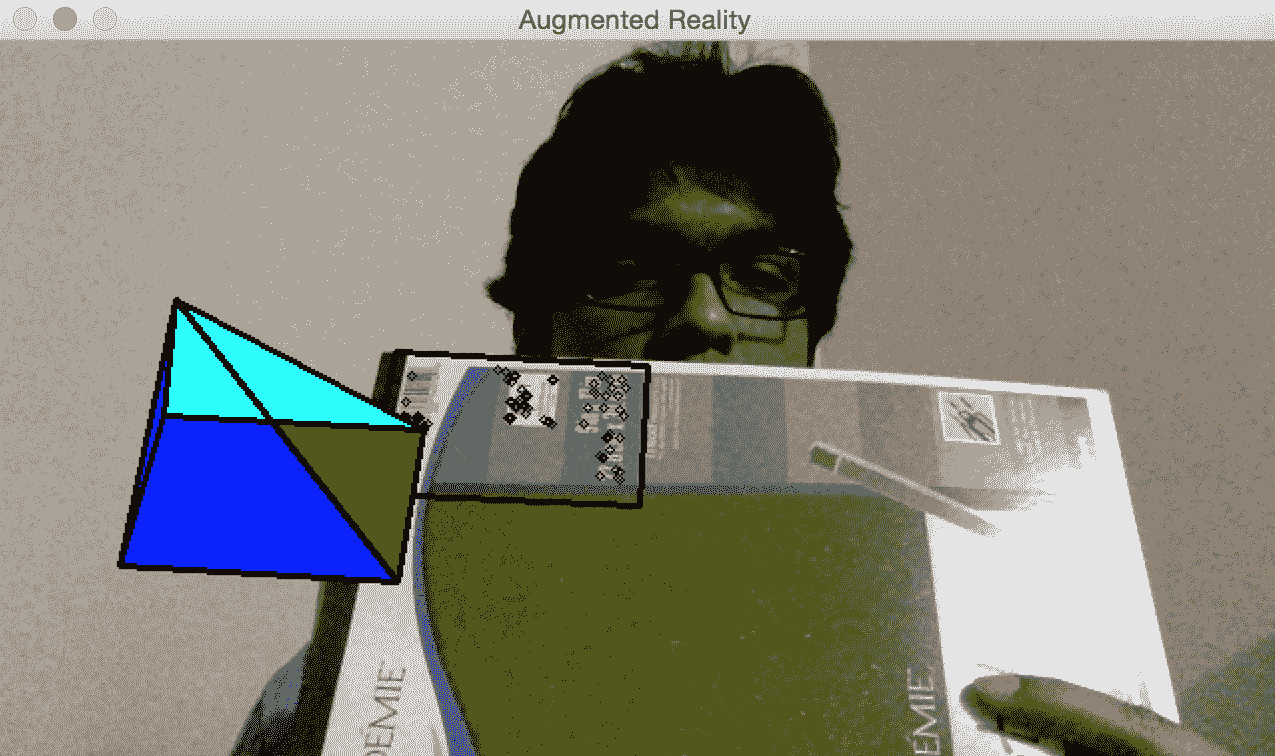# 十、增强现实

• 增强现实的前提
• 什么是姿势估计
• 如何追踪平面对象
• 如何将坐标从 3D 映射到 2D
• 如何在视频上实时叠加图形

# 增强现实系统是什么样的？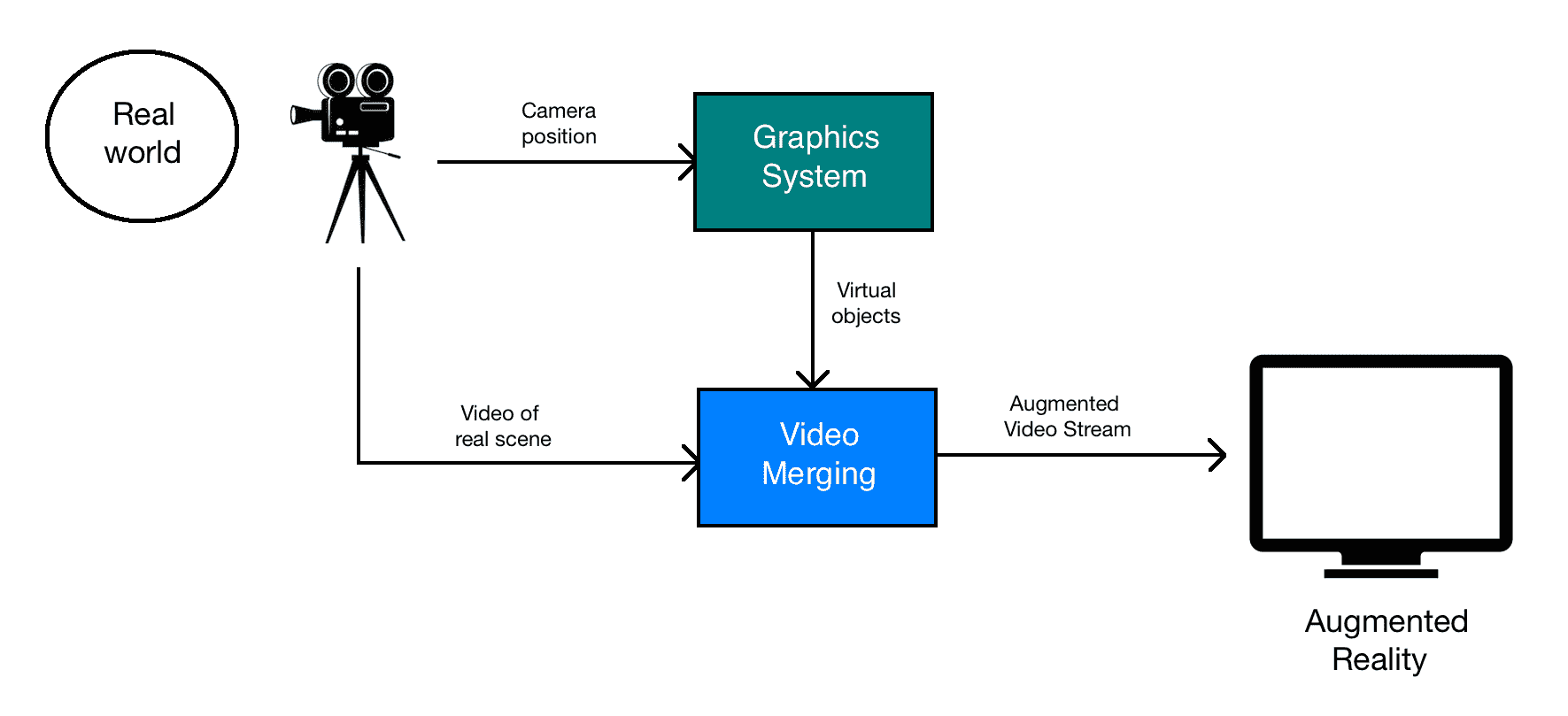# 增强现实的几何变换

• 对象到场景：此转换是指转换虚拟对象的 3D 坐标，并在我们真实世界场景的坐标框中表达它们。 这样可以确保我们将虚拟对象放置在正确的位置。
• 场景到摄像机：此转换是指现实世界中摄像机的姿势。 通过姿势，我们表示摄像机的方向和位置。 我们需要估计摄像机的视点，以便我们知道如何覆盖虚拟对象。
• 摄像机到图像：这是指摄像机的校准参数。 这定义了我们如何将 3D 对象投影到 2D 图像平面上。 这是我们最终将实际看到的图像。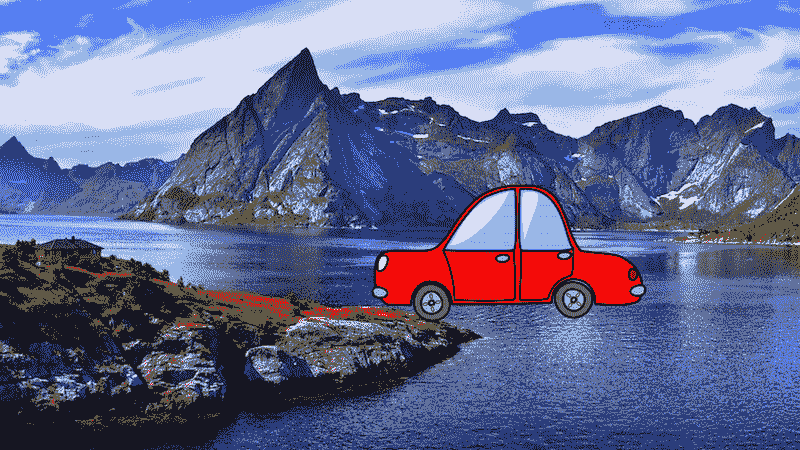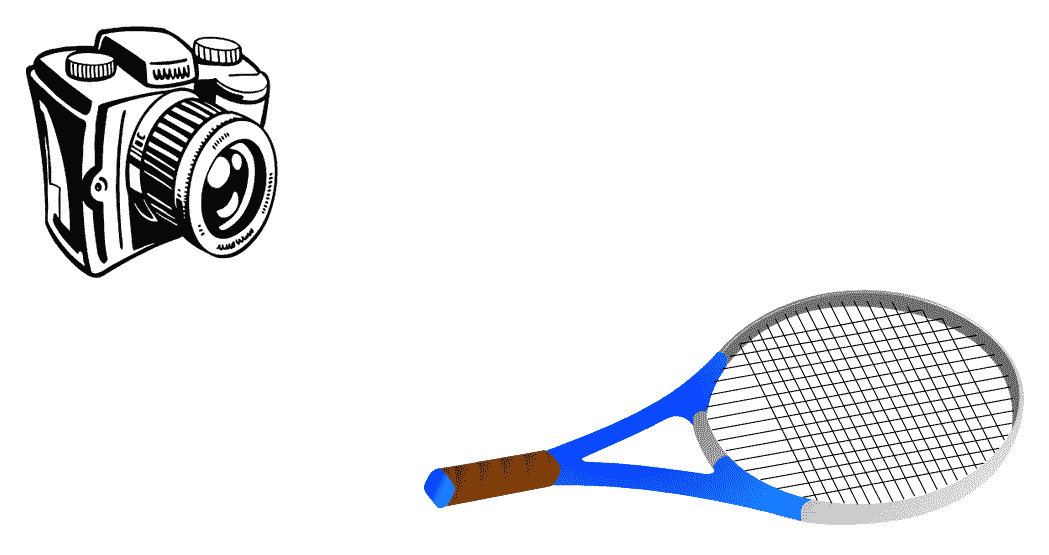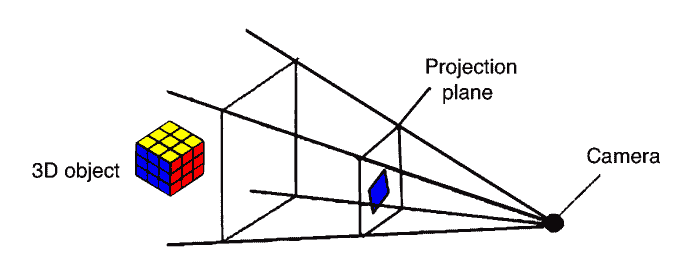# 什么是姿势估计？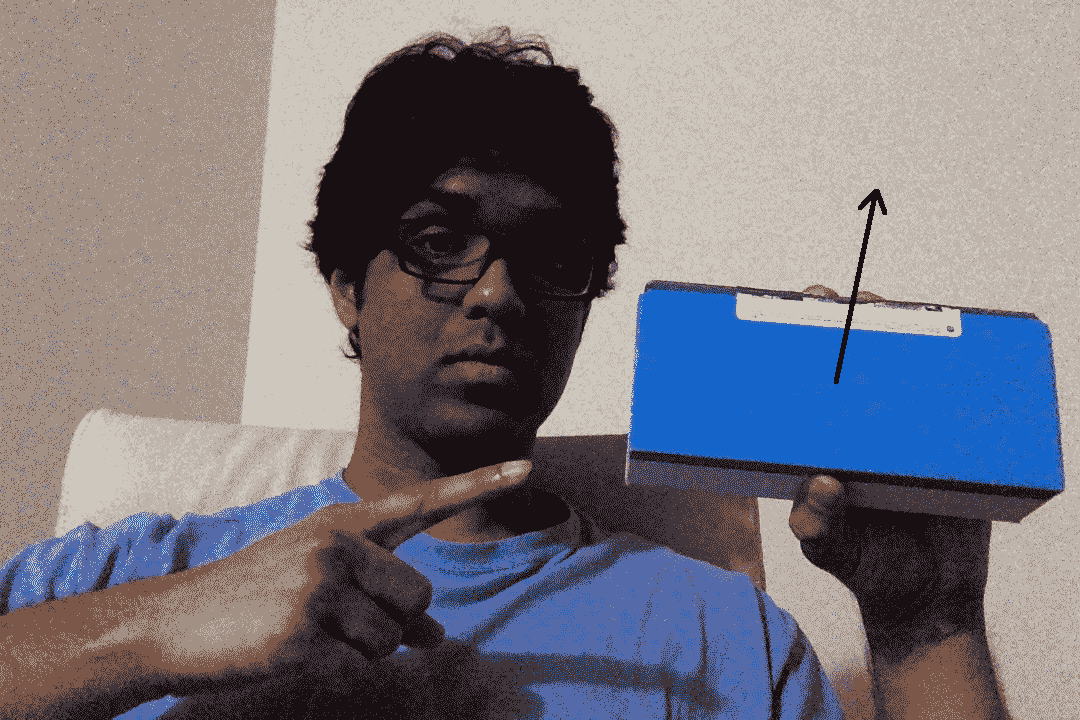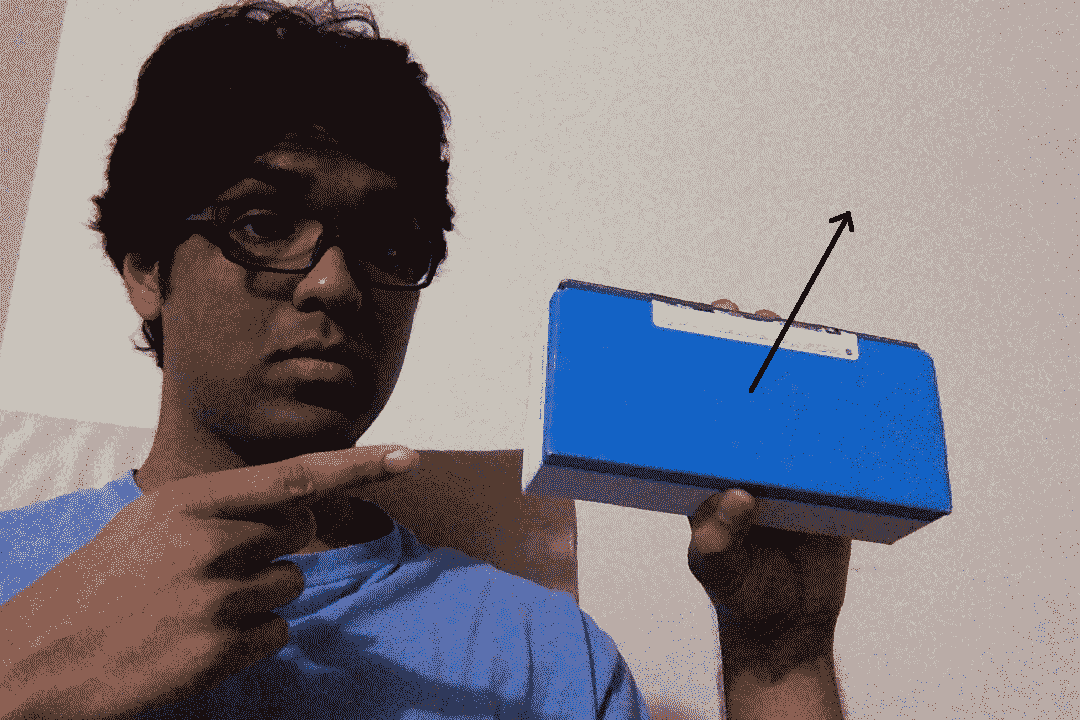# 如何追踪平面对象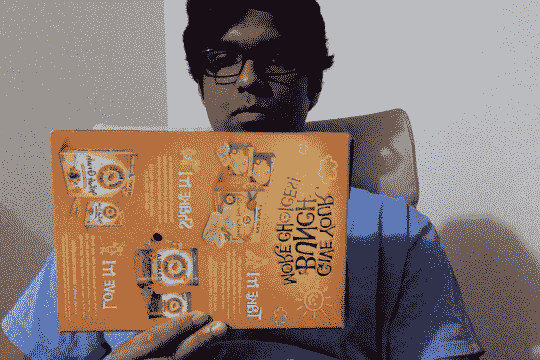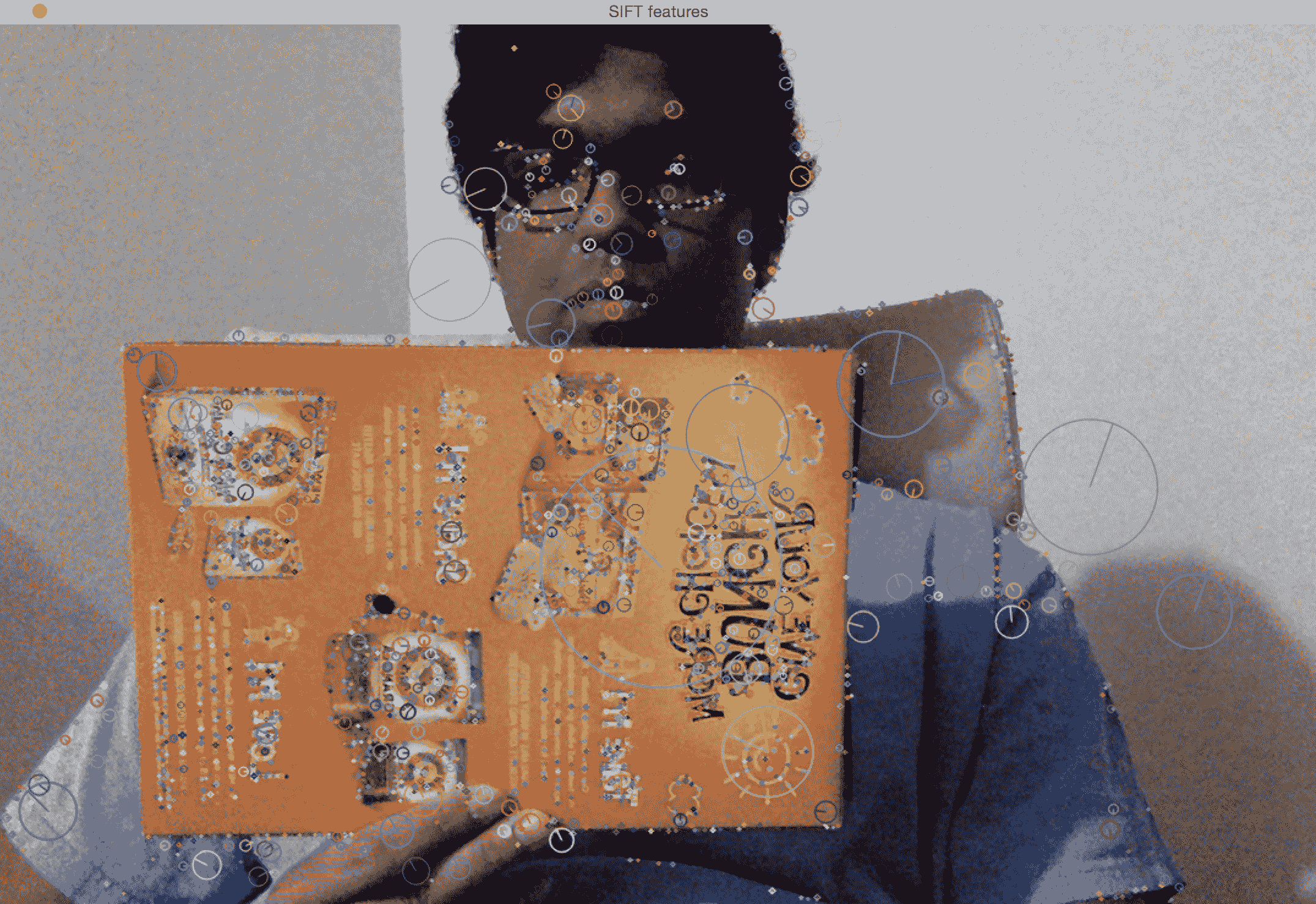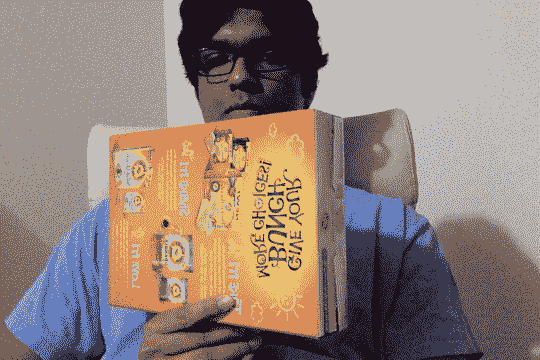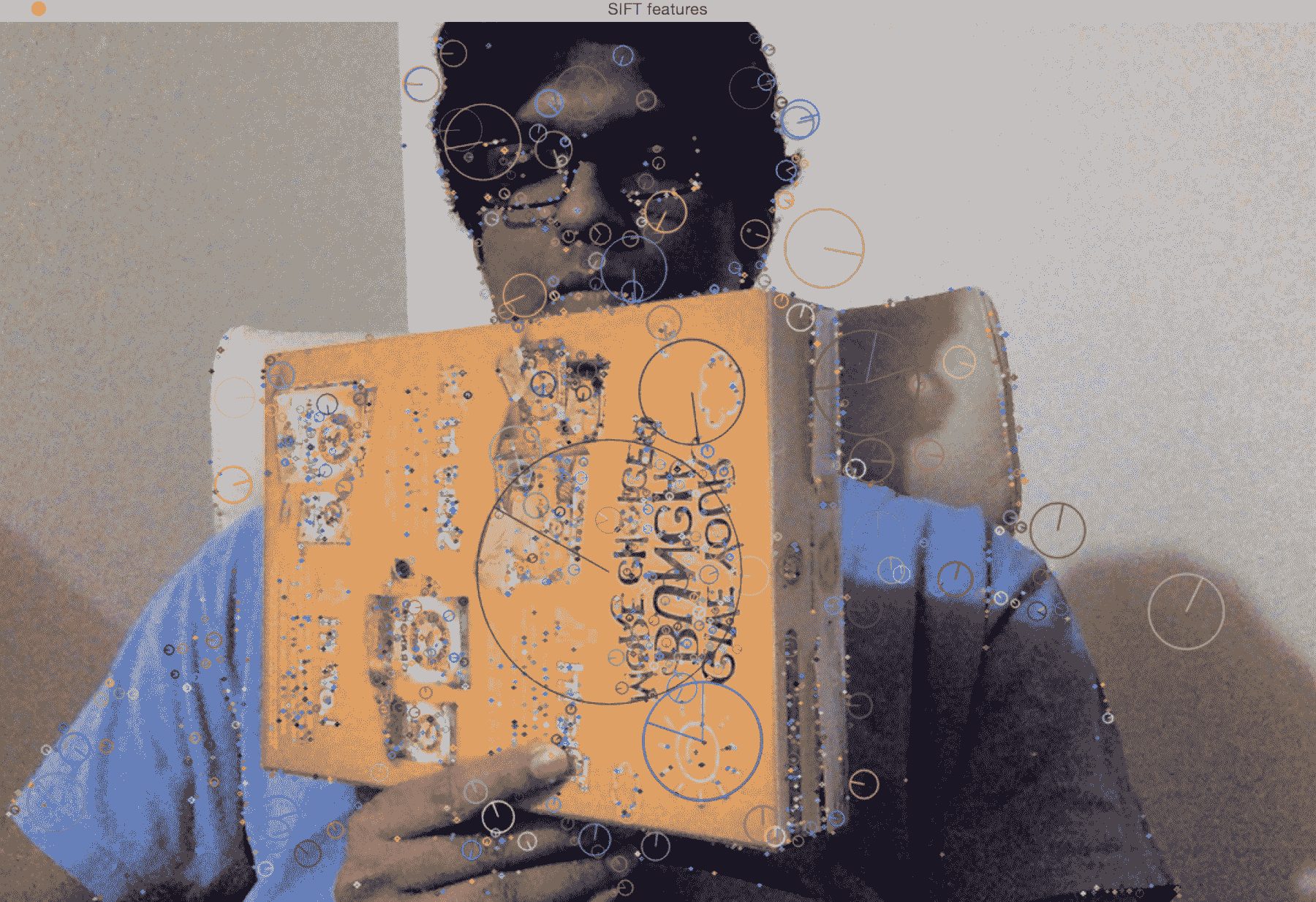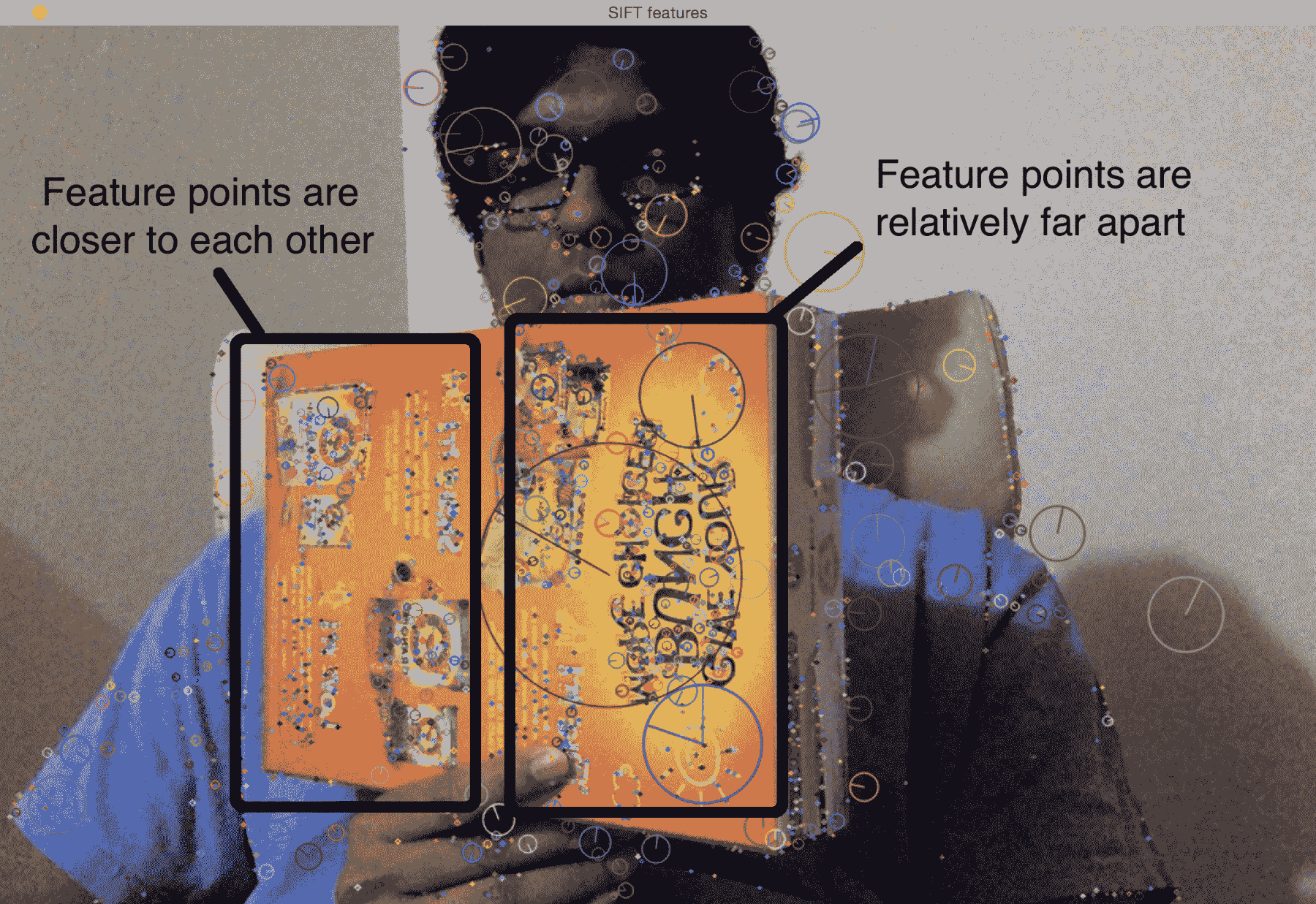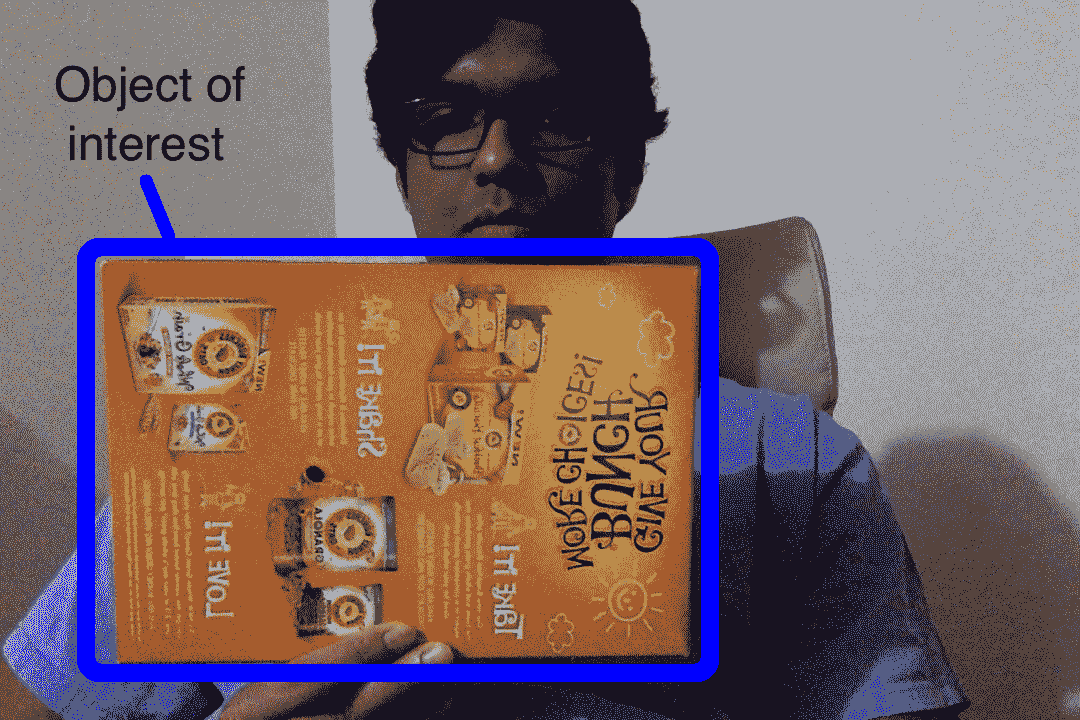class ROISelector(object):
def __init__(self, win_name, init_frame, callback_func):
self.callback_func = callback_func
self.selected_rect = None
self.drag_start = None
self.tracking_state = 0
event_params = {"frame": init_frame}
cv2.namedWindow(win_name)
cv2.setMouseCallback(win_name, self.mouse_event, event_params)

def mouse_event(self, event, x, y, flags, param):
x, y = np.int16([x, y])

# Detecting the mouse button down event
if event == cv2.EVENT_LBUTTONDOWN:
self.drag_start = (x, y)
self.tracking_state = 0

if self.drag_start:
if event == cv2.EVENT_MOUSEMOVE:
h, w = param["frame"].shape[:2]
xo, yo = self.drag_start
x0, y0 = np.maximum(0, np.minimum([xo, yo], [x, y]))
x1, y1 = np.minimum([w, h], np.maximum([xo, yo], [x, y]))
self.selected_rect = None

if x1-x0 > 0 and y1-y0 > 0:
self.selected_rect = (x0, y0, x1, y1)

elif event == cv2.EVENT_LBUTTONUP:
self.drag_start = None
if self.selected_rect is not None:
self.callback_func(self.selected_rect)
self.selected_rect = None
self.tracking_state = 1

def draw_rect(self, img, rect):
if not rect: return False
x_start, y_start, x_end, y_end = rect
cv2.rectangle(img, (x_start, y_start), (x_end, y_end), (0, 255, 0), 2)
return True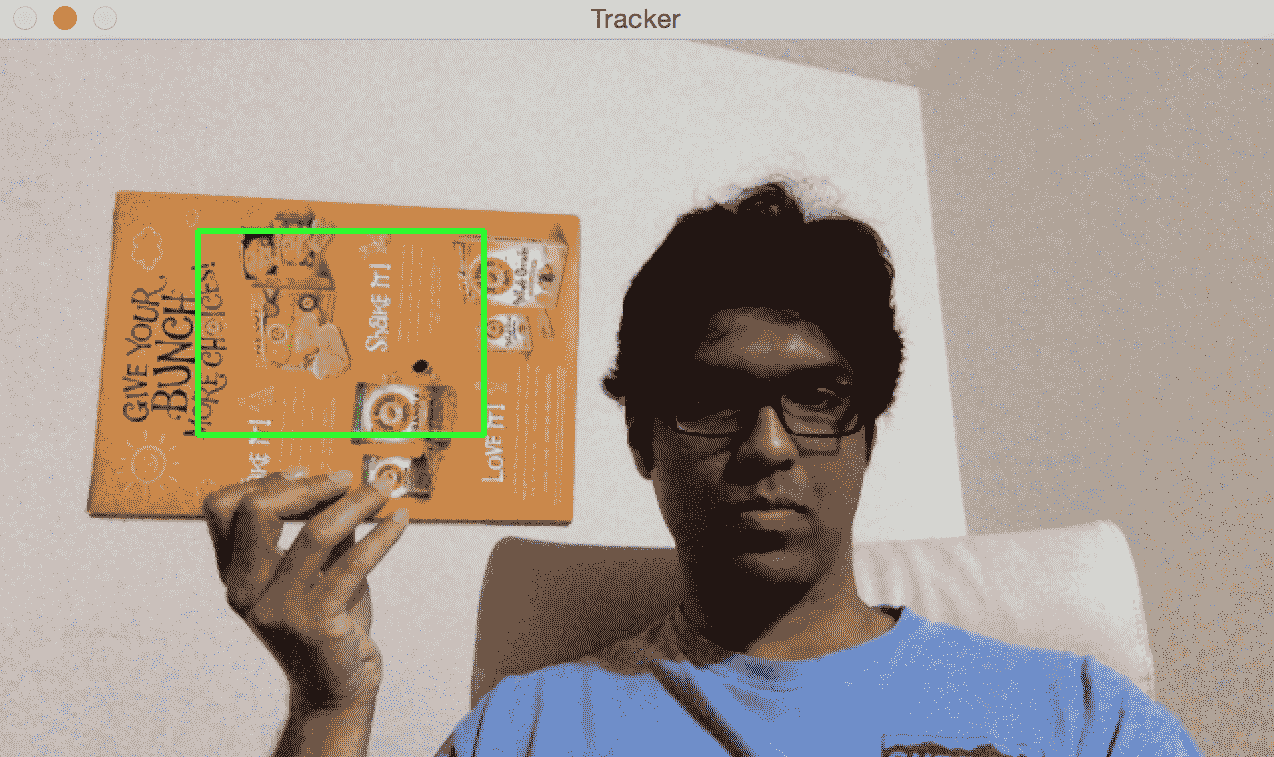PoseEstimator类将从其方法add_target()中获得兴趣区域，并从它们中提取这些特征点，这将使我们能够跟踪物体的运动：

class PoseEstimator(object):
def __init__(self):
# Use locality sensitive hashing algorithm
flann_params = dict(algorithm = 6, table_number = 6, key_size = 12, multi_probe_level = 1)

self.min_matches = 10
self.cur_target = namedtuple('Current', 'image, rect, keypoints, descriptors, data')
self.tracked_target = namedtuple('Tracked', 'target, points_prev, points_cur, H, quad')

self.feature_detector = cv2.ORB_create()
self.feature_detector.setMaxFeatures(1000)
self.feature_matcher = cv2.FlannBasedMatcher(flann_params, {})
self.tracking_targets = []

# Function to add a new target for tracking
x_start, y_start, x_end, y_end = rect
keypoints, descriptors = [], []
for keypoint, descriptor in zip(*self.detect_features(image)):
x, y = keypoint.pt
if x_start <= x <= x_end and y_start <= y <= y_end:
keypoints.append(keypoint)
descriptors.append(descriptor)

descriptors = np.array(descriptors, dtype='uint8')
target = self.cur_target(image=image, rect=rect, keypoints=keypoints, descriptors=descriptors, data=None)
self.tracking_targets.append(target)

# To get a list of detected objects
def track_target(self, frame):
self.cur_keypoints, self.cur_descriptors = self.detect_features(frame)

if len(self.cur_keypoints) < self.min_matches: return []
try: matches = self.feature_matcher.knnMatch(self.cur_descriptors, k=2)
except Exception as e:
print('Invalid target, please select another with features to extract')
return []
matches = [match for match in matches if len(match) == 2 and match.distance < match.distance * 0.75]
if len(matches) < self.min_matches: return []

matches_using_index = [[] for _ in range(len(self.tracking_targets))]
for match in matches:
matches_using_index[match.imgIdx].append(match)

tracked = []
for image_index, matches in enumerate(matches_using_index):
if len(matches) < self.min_matches: continue

target = self.tracking_targets[image_index]
points_prev = [target.keypoints[m.trainIdx].pt for m in matches]
points_cur = [self.cur_keypoints[m.queryIdx].pt for m in matches]
points_prev, points_cur = np.float32((points_prev, points_cur))
H, status = cv2.findHomography(points_prev, points_cur, cv2.RANSAC, 3.0)
status = status.ravel() != 0

if status.sum() < self.min_matches: continue

points_prev, points_cur = points_prev[status], points_cur[status]

x_start, y_start, x_end, y_end = target.rect
quad = np.float32([[x_start, y_start], [x_end, y_start], [x_end, y_end], [x_start, y_end]])
tracked.append(track)

tracked.sort(key = lambda x: len(x.points_prev), reverse=True)
return tracked

# Detect features in the selected ROIs and return the keypoints and descriptors
def detect_features(self, frame):
keypoints, descriptors = self.feature_detector.detectAndCompute(frame, None)
if descriptors is None: descriptors = []
return keypoints, descriptors

# Function to clear all the existing targets
def clear_targets(self):
self.feature_matcher.clear()
self.tracking_targets = []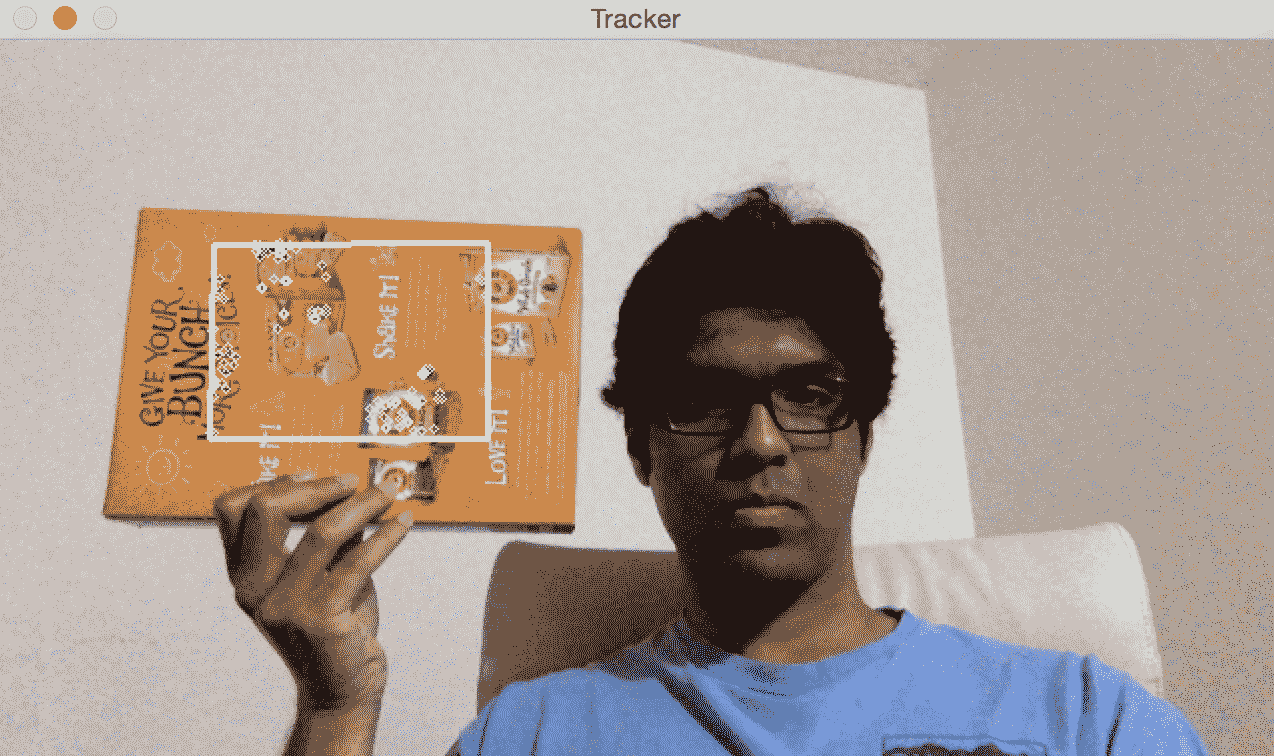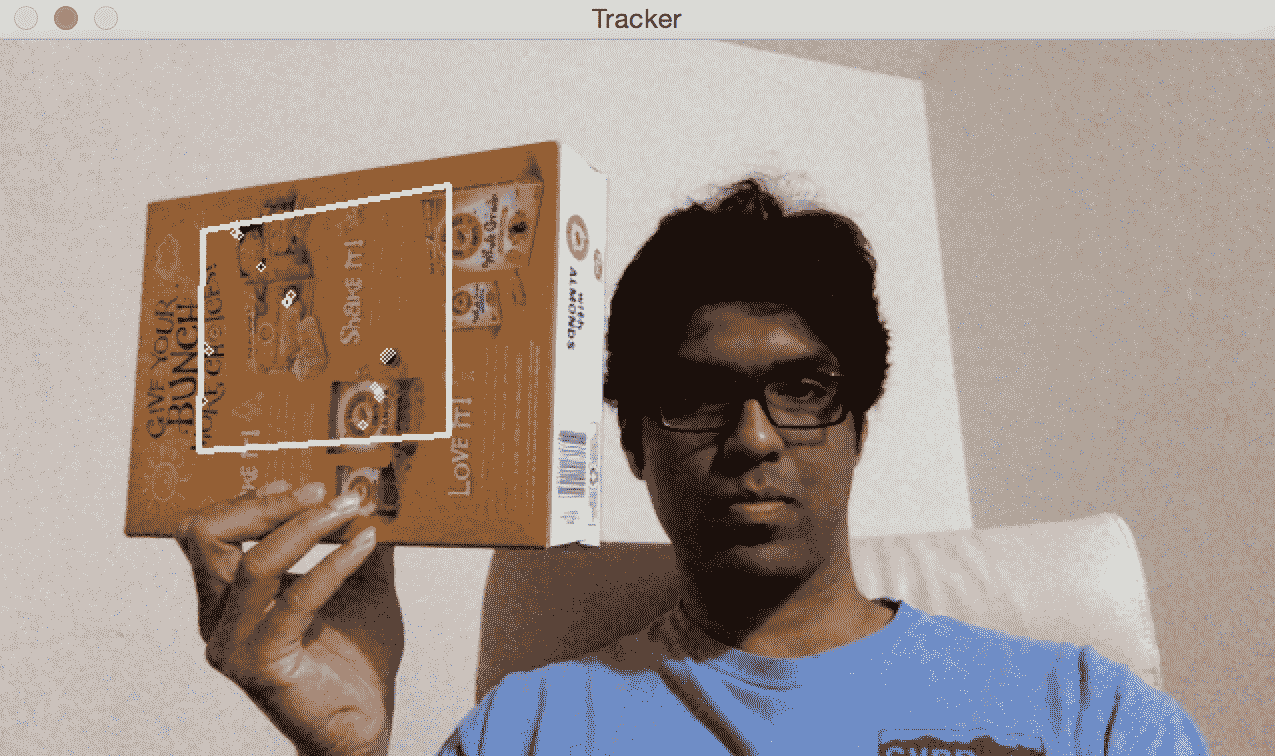import sys
from collections import namedtuple

import cv2
import numpy as np

class VideoHandler(object):
def __init__(self, capId, scaling_factor, win_name):
self.cap = cv2.VideoCapture(capId)
self.pose_tracker = PoseEstimator()
self.win_name = win_name
self.scaling_factor = scaling_factor

self.rect = None
self.frame = cv2.resize(frame, None, fx=scaling_factor, fy=scaling_factor, interpolation=cv2.INTER_AREA)
self.roi_selector = ROISelector(win_name, self.frame, self.set_rect)

def set_rect(self, rect):
self.rect = rect

def start(self):
paused = False
while True:
if not paused or self.frame is None:
scaling_factor = self.scaling_factor
frame = cv2.resize(frame, None, fx=scaling_factor, fy=scaling_factor, interpolation=cv2.INTER_AREA)
if not ret: break
self.frame = frame.copy()

img = self.frame.copy()
if not paused and self.rect is not None:
tracked = self.pose_tracker.track_target(self.frame)
for item in tracked:
cv2.polylines(img, [np.int32(item.quad)], True, (255, 255, 255), 2)
for (x, y) in np.int32(item.points_cur):
cv2.circle(img, (x, y), 2, (255, 255, 255))

self.roi_selector.draw_rect(img, self.rect)
cv2.imshow(self.win_name, img)
ch = cv2.waitKey(1)
if ch == ord(' '): paused = not paused
if ch == ord('c'): self.pose_tracker.clear_targets()
if ch == 27: break

if __name__ == '__main__':
VideoHandler(0, 0.8, 'Tracker').start()


# 代码内部发生了什么？

track_target方法处理所有跟踪。 我们采用当前框架并提取所有关键点。 但是，我们对视频当前帧中的所有关键点并不真正感兴趣。 我们只想要属于我们目标对象的关键点。 因此，现在我们的工作是在当前帧中找到最接近的关键点。

# 将坐标从 3D 映射到 2D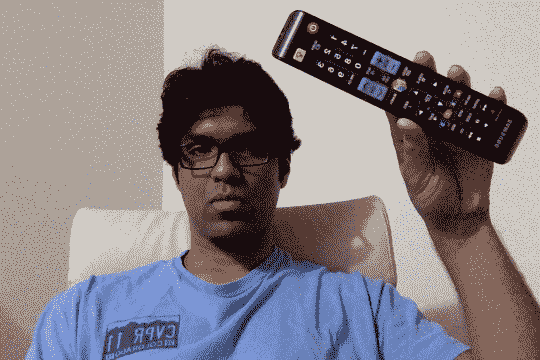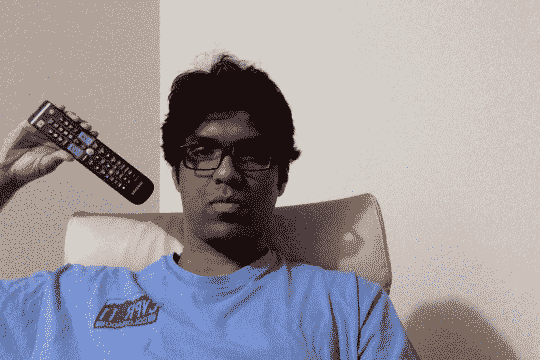solvePnP(InputArray objectPoints, InputArray imagePoints, InputArray cameraMatrix, InputArray distCoeffs, OutputArray rvec, OutputArray tvec, bool useExtrinsicGuess, int flags)


# 如何在视频上叠加 3D 对象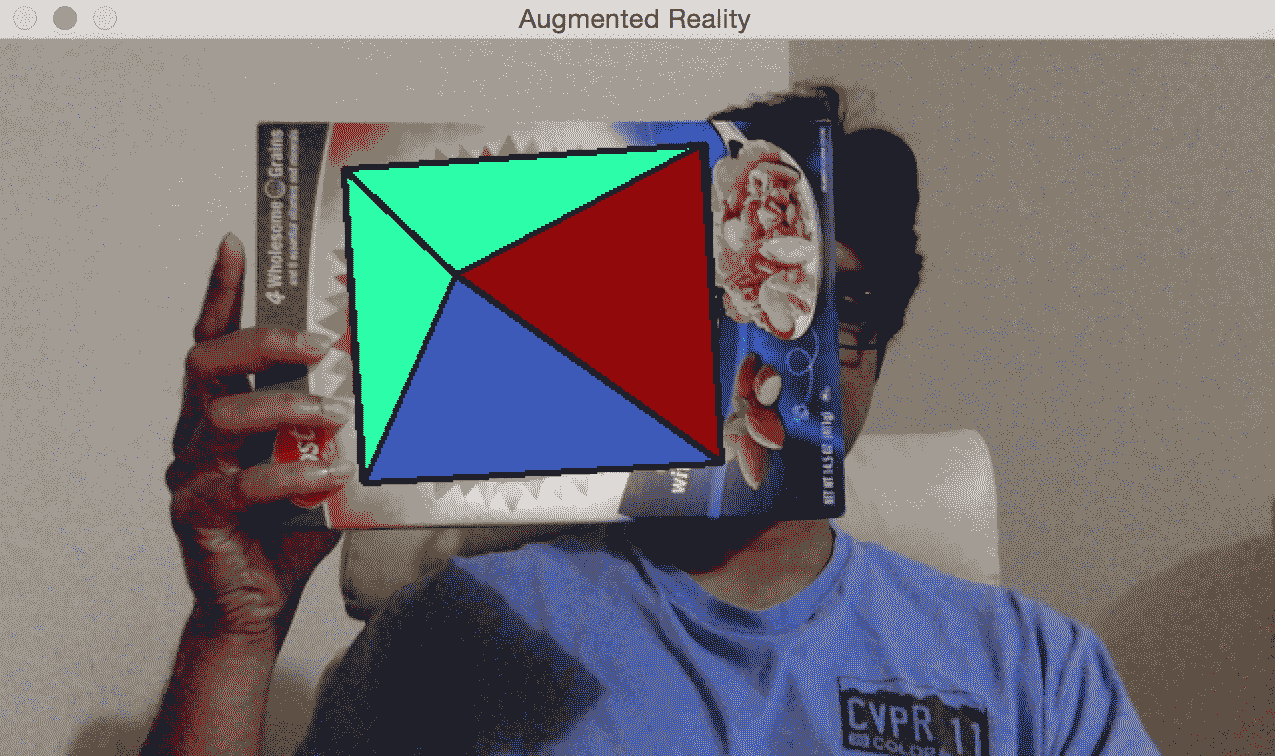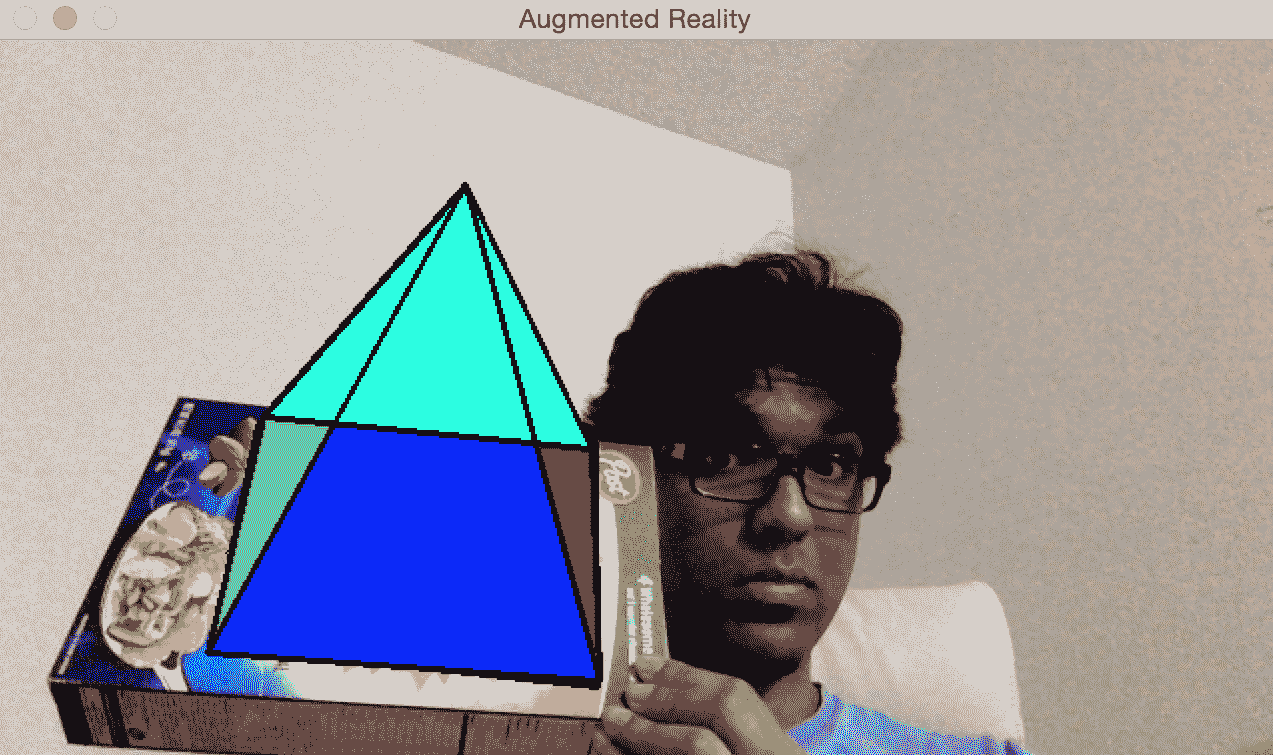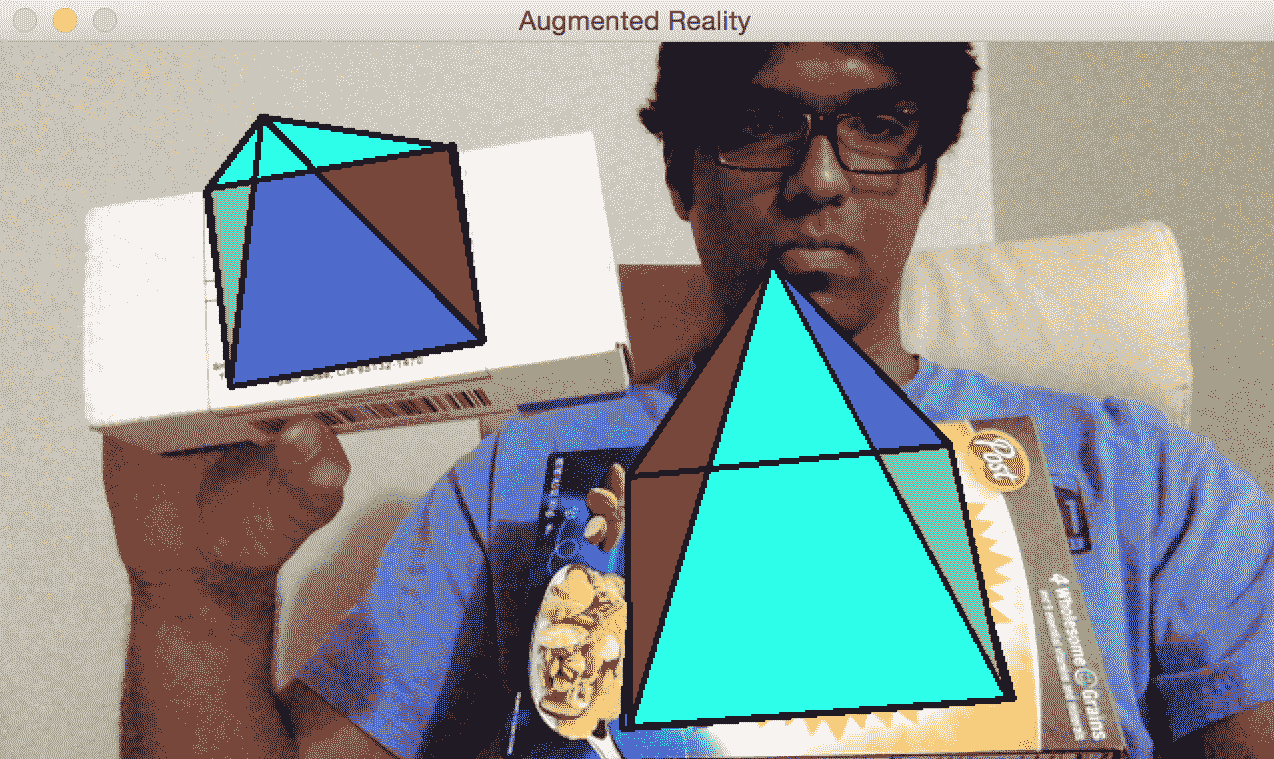import cv2
import numpy as np

from pose_estimation import PoseEstimator, ROISelector

class Tracker(object):
def __init__(self, capId, scaling_factor, win_name):
self.cap = cv2.VideoCapture(capId)
self.rect = None
self.win_name = win_name
self.scaling_factor = scaling_factor
self.tracker = PoseEstimator()

self.rect = None
self.frame = cv2.resize(frame, None, fx=scaling_factor, fy=scaling_factor, interpolation=cv2.INTER_AREA)

self.roi_selector = ROISelector(win_name, self.frame, self.set_rect)
self.overlay_vertices = np.float32([[0, 0, 0], [0, 1, 0], [1, 1, 0], [1, 0, 0], [0.5, 0.5, 4]])
self.overlay_edges = [(0, 1), (1, 2), (2, 3), (3, 0), (0,4), (1,4), (2,4), (3,4)]
self.color_base = (0, 255, 0)
self.color_lines = (0, 0, 0)

def set_rect(self, rect):
self.rect = rect

def start(self):
paused = False
while True:
if not paused or self.frame is None:
scaling_factor = self.scaling_factor
frame = cv2.resize(frame, None, fx=scaling_factor, fy=scaling_factor,\
interpolation=cv2.INTER_AREA)
if not ret: break

self.frame = frame.copy()

img = self.frame.copy()
if not paused:
tracked = self.tracker.track_target(self.frame)
for item in tracked:
True, self.color_lines, 2)
for (x, y) in np.int32(item.points_cur):
cv2.circle(img, (x, y), 2,
self.color_lines)

self.overlay_graphics(img, item)

self.roi_selector.draw_rect(img, self.rect)
cv2.imshow(self.win_name, img)
ch = cv2.waitKey(1)
if ch == ord(' '): self.paused = not self.paused
if ch == ord('c'): self.tracker.clear_targets()
if ch == 27: break

def overlay_graphics(self, img, tracked):
x_start, y_start, x_end, y_end = tracked.target.rect
quad_3d = np.float32([[x_start, y_start, 0], [x_end,
y_start, 0],
[x_end, y_end, 0], [x_start, y_end, 0]])
h, w = img.shape[:2]
K = np.float64([[w, 0, 0.5*(w-1)],
[0, w, 0.5*(h-1)],
[0, 0, 1.0]])
dist_coef = np.zeros(4)
cameraMatrix=K, distCoeffs=dist_coef)
verts = self.overlay_vertices * \
[(x_end-x_start), (y_end-y_start), -(x_end-x_start)*0.3] + (x_start, y_start, 0)
verts = cv2.projectPoints(verts, rvec, tvec, cameraMatrix=K, distCoeffs=dist_coef).reshape(-1, 2)

verts_floor = np.int32(verts).reshape(-1,2)
cv2.drawContours(img, contours=[verts_floor[:4]], contourIdx=-1, color=self.color_base, thickness=-3)
cv2.drawContours(img, contours=[np.vstack((verts_floor[:2], verts_floor[4:5]))], contourIdx=-1, color=(0,255,0), thickness=-3)
cv2.drawContours(img, contours=[np.vstack((verts_floor[1:3], verts_floor[4:5]))], contourIdx=-1, color=(255,0,0), thickness=-3)
cv2.drawContours(img, contours=[np.vstack((verts_floor[2:4], verts_floor[4:5]))], contourIdx=-1, color=(0,0,150), thickness=-3)
cv2.drawContours(img, contours=[np.vstack((verts_floor[3:4], verts_floor[0:1], verts_floor[4:5]))], contourIdx=-1, color=(255,255,0), thickness=-3)

for i, j in self.overlay_edges:
(x_start, y_start), (x_end, y_end) = verts[i], verts[j]
cv2.line(img, (int(x_start), int(y_start)), (int(x_end), int(y_end)), self.color_lines, 2)

if __name__ == '__main__':
Tracker(0, 0.8, 'Augmented Reality').start()


# 让我们看一下代码

Tracker类用于执行此处的所有计算。 我们使用通过边和顶点定义的金字塔结构初始化该类。 我们用来跟踪表面的逻辑与我们之前讨论的相同，因为我们使用的是同一类。 我们只需要使用solvePnPprojectPoints将 3D 金字塔映射到 2D 曲面即可。

# 让我们添加一些动作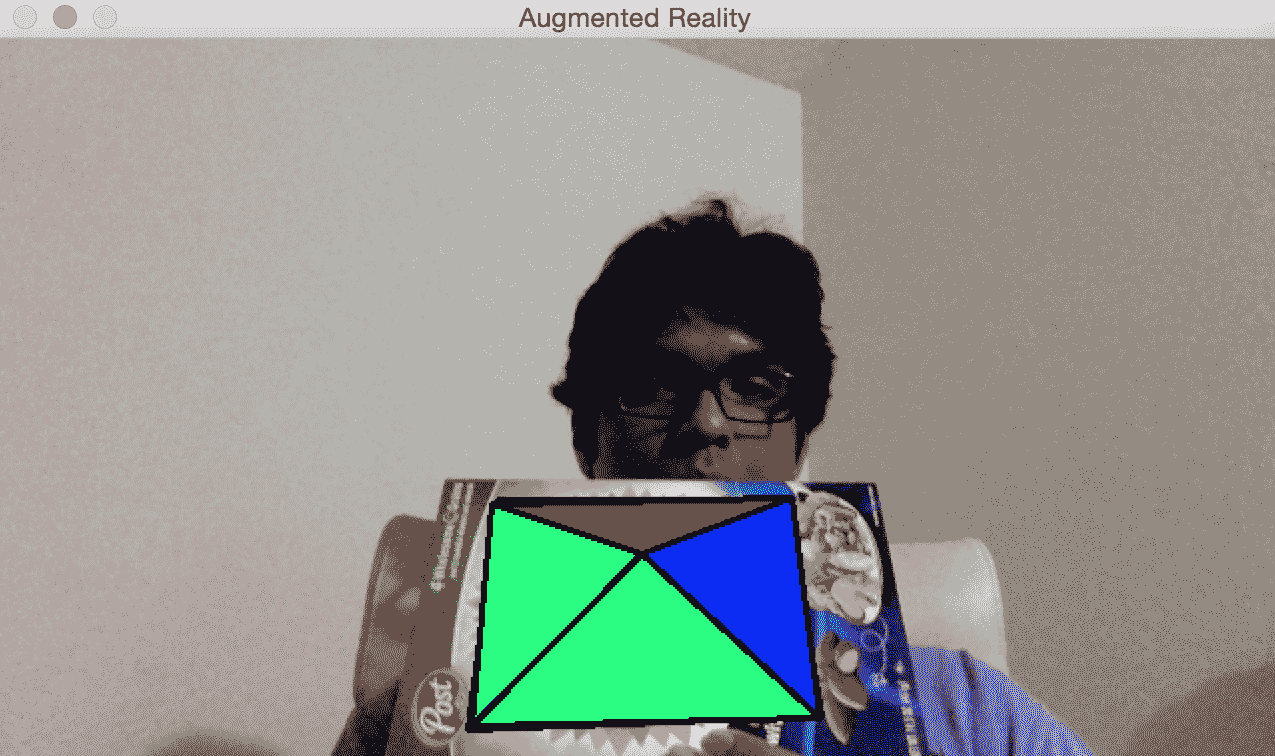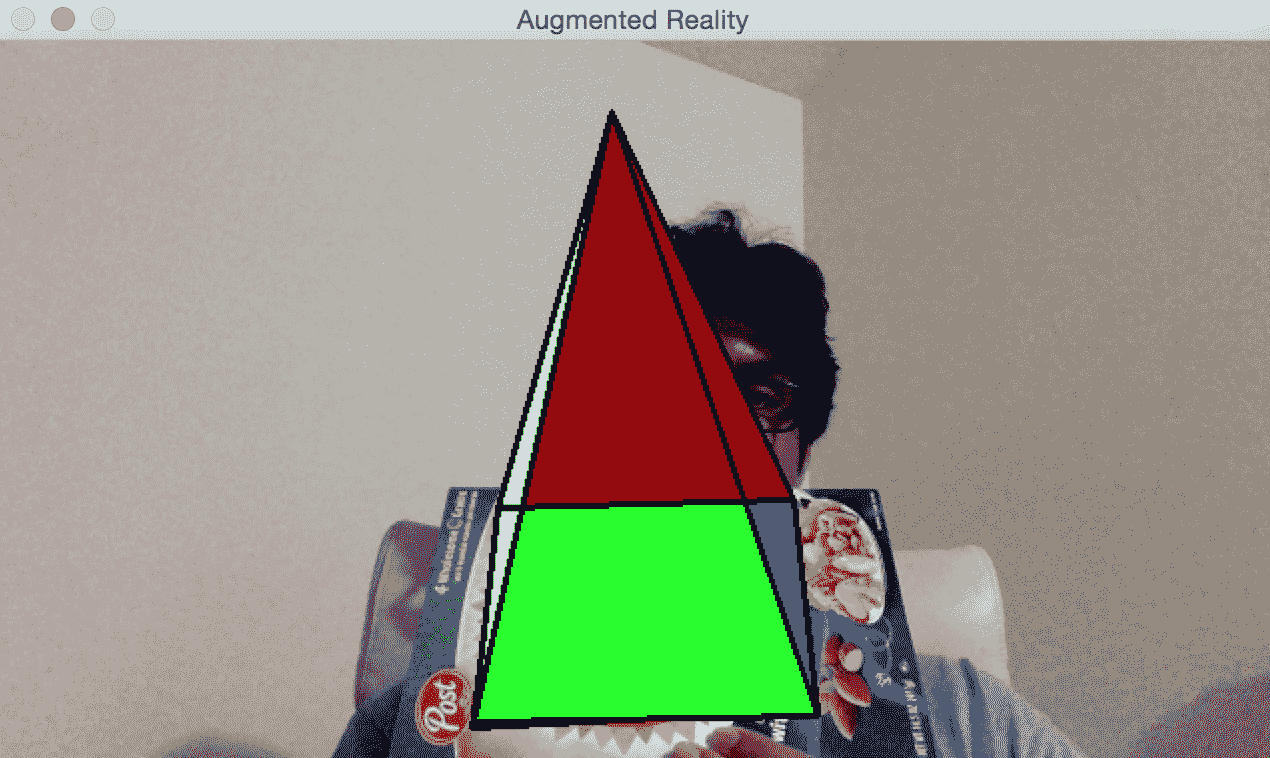self.overlay_vertices = np.float32([[0, 0, 0], [0, 1, 0], [1, 1, 0], [1, 0, 0], [0.5, 0.5, 4]])
self.overlay_edges = [(0, 1), (1, 2), (2, 3), (3, 0),
(0,4), (1,4), (2,4), (3,4)]
self.color_base = (0, 255, 0)
self.color_lines = (0, 0, 0)

self.graphics_counter = 0
self.time_counter = 0


    def overlay_graphics(self, img, tracked):
x_start, y_start, x_end, y_end = tracked.target.rect
quad_3d = np.float32([[x_start, y_start, 0], [x_end,
y_start, 0],
[x_end, y_end, 0], [x_start, y_end, 0]])
h, w = img.shape[:2]
K = np.float64([[w, 0, 0.5*(w-1)],
[0, w, 0.5*(h-1)],
[0, 0, 1.0]])
dist_coef = np.zeros(4)
cameraMatrix=K, distCoeffs=dist_coef)
verts = self.overlay_vertices * \
[(x_end-x_start), (y_end-y_start), -(x_end-x_start)*0.3] + (x_start, y_start, 0)
verts = cv2.projectPoints(verts, rvec, tvec, cameraMatrix=K,
distCoeffs=dist_coef).reshape(-1, 2)

verts_floor = np.int32(verts).reshape(-1,2)
cv2.drawContours(img, contours=[verts_floor[:4]],
contourIdx=-1, color=self.color_base, thickness=-3)
cv2.drawContours(img, contours=[np.vstack((verts_floor[:2],
verts_floor[4:5]))], contourIdx=-1, color=(0,255,0), thickness=-3)
cv2.drawContours(img, contours=[np.vstack((verts_floor[1:3],
verts_floor[4:5]))], contourIdx=-1, color=(255,0,0), thickness=-3)
cv2.drawContours(img, contours=[np.vstack((verts_floor[2:4],
verts_floor[4:5]))], contourIdx=-1, color=(0,0,150), thickness=-3)
cv2.drawContours(img, contours=[np.vstack((verts_floor[3:4],
verts_floor[0:1], verts_floor[4:5]))], contourIdx=-1, color=(255,255,0),thickness=-3)

for i, j in self.overlay_edges:
(x_start, y_start), (x_end, y_end) = verts[i], verts[j]
cv2.line(img, (int(x_start), int(y_start)), (int(x_end), int(y_end)),
self.color_lines, 2)